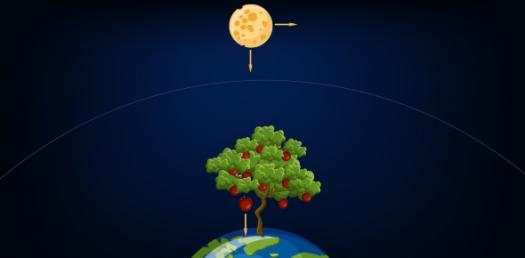# Gravitation Quiz

10 Questions | Attempts: 6121
ShareSettingsThis is gravitation quiz. No searchs from google

• 1.
What quantity is gravitational force ?
• A.

Scalar quantity

• B.

Vector quantity

• C.

A and B both

• D.

None

• 2.
Which one of the following is true ?
• A.

Gravitational force is indirectly proportional to masses of two objects

• B.

Gravitational force is directly proportional to square of distance between two bodies

• C.

Gravitational force is directly proportional to masses of two bodies

• D.

None

• E.

A , b , c all

• 3.
Unit of G is ?
• A.

N m2 kg2

• B.

N m/kg

• C.

N m2 / kg2

• D.

N kg2/m2

• 4.
Gravitational acceleration does not depend on ?
• A.

Mass of the object

• B.

Weight of the object

• C.

Gravitation constant

• D.

Distance from earth surface

• 5.
Where is the value of Gravitational acceleration is minimum ?
• A.

Alps - Europe

• B.

Equator

• C.

Poles

• D.

Tropic of cancer

• E.

Tropic of capricorn

• 6.
What is the value of Gravitational acceleration on the surface of the moon ?
• A.

9.8 m/s2

• B.

33 cm/s2

• C.

1.608 m/s2

• D.

15 m/s2

• 7.
What will be the mass of the object on moon surface whose mass is 60 kg on earths surface
• A.

60 kg

• B.

10 kg

• C.

60 N

• D.

10 N

• 8.
What is the unit gravitational force ?
• A.

Newton

• B.

Kelvin

• C.

Kg

• D.

Metre

• 9.
What is the weight of an object  on earths surfacewhich weighs 60 N on moon's surface ?
• A.

360 N

• B.

10 N

• C.

60 kg

• D.

2 kg

• 10.
Weight depends on which two factors ?
• A.

Mass and energy

• B.

Mass and gravitation constant

• C.

Mass and Gravitational acceleration

• D.

Above a, b,c

• E.

None

## Related TopicsBack to top
×

Wait!
Here's an interesting quiz for you.